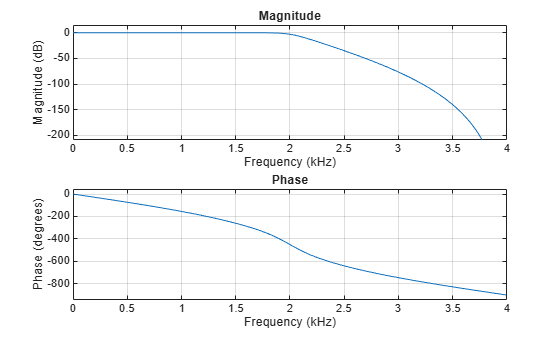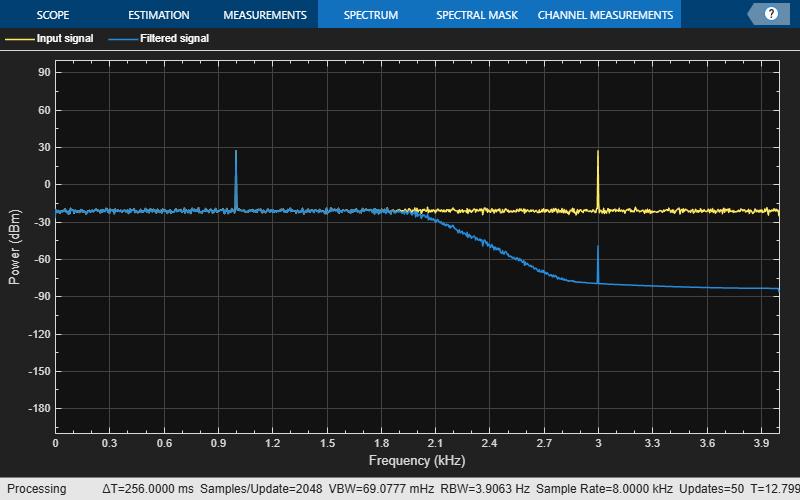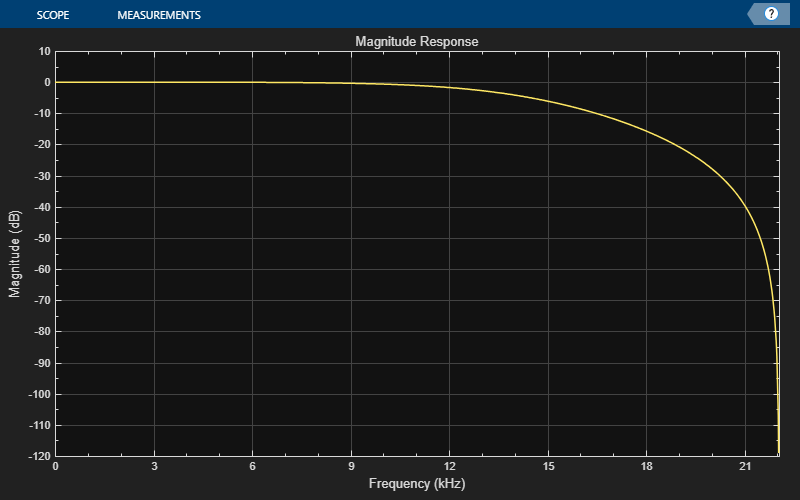# dsp.SOSFilter

Second-order section biquadratic IIR filter structures

## Description

The `dsp.SOSFilter` System object™ implements an IIR filter structure using second-order sections (SOS).

To implement an IIR filter structure using SOS:

1. Create the `dsp.SOSFilter` object and set its properties.

2. Call the object with arguments, as if it were a function.

## Creation

### Syntax

``sos = dsp.SOSFilter``
``sos = dsp.SOSFilter(num,den)``
``sos = dsp.SOSFilter(Name,Value)``

### Description

example

````sos = dsp.SOSFilter` returns a biquadratic IIR filter System object, `sos`, which independently filters each channel (column) of the input over time using a specified biquadratic structure.```
````sos = dsp.SOSFilter(num,den)` returns a biquadratic filter object with the `Numerator` property set to `num` and the `Denominator` property set to `den`.```
````sos = dsp.SOSFilter(Name,Value)` returns a biquadratic filter object with each property set to the specified value. Enclose each property name in single quotes.Example: ```sos = dsp.SOSFilter('CoefficientSource','Input port')``````

## Properties

expand all

Unless otherwise indicated, properties are nontunable, which means you cannot change their values after calling the object. Objects lock when you call them, and the `release` function unlocks them.

If a property is tunable, you can change its value at any time.

Filter structure, specified as one of `'Direct form I'`, `'Direct form I transposed'`, `'Direct form II'`, or `'Direct form II transposed'`.

Source of the filter coefficients, specified as one of the following:

• `'Property'` –– The filter coefficients are specified through the `Numerator`, `Denominator`, and `ScaleValues` properties.

• `'Input port'` –– The numerator coefficients, denominator coefficients, and the scale values are specified as inputs to the object while running the algorithm. For more details, see Usage.

Numerator coefficients of the filter, specified as an N-by-3 matrix, where N is the number of biquadratic sections.

The size of this property cannot be modified once you have run the System object algorithm. However, the coefficient values can change as the property is tunable.

Tunable: Yes

#### Dependencies

To enable this property, set the `CoefficientSource` property to `'Property'`.

Data Types: `single` | `double` | `int8` | `int16` | `int32` | `int64` | `uint8` | `uint16` | `uint32` | `uint64`
Complex Number Support: Yes

Denominator coefficients of the filter, specified as an N-by-3 matrix, where N is the number of biquadratic sections.

The leading denominator coefficient is always assumed to be 1. If any other value is specified in the first column, the object ignores this value and treats it as 1.

The size of this property cannot be modified once you step through the algorithm. However, the denominator values can be modified as the property is tunable.

Tunable: Yes

#### Dependencies

To enable this property, set `CoefficientSource` property to `'Property'`.

Data Types: `single` | `double` | `int8` | `int16` | `int32` | `int64` | `uint8` | `uint16` | `uint32` | `uint64`
Complex Number Support: Yes

Specify if the filter has scale values for each section. When set to `true`, using the `ScaleValues` property, you can specify the scale values to be applied before and after each section of the biquadratic filter.

Scale values to apply before and after each section of a biquadratic filter, specified as a vector. The length of the `ScaleValues` vector must be N + 1, where N is the number of biqaudratic sections. If you set this property to a scalar value, the scalar value is used as the gain value only before the first filter section. The remaining gain values are set to `1`. If you set this property to a vector of N + 1 values, each value is used for a separate section of the filter.

Tunable: Yes

#### Dependencies

This property applies only when you set the `CoefficientSource` property to `'Property'` and `HasScaleValues` property to `true`.

Data Types: `single` | `double` | `int8` | `int16` | `int32` | `int64` | `uint8` | `uint16` | `uint32` | `uint64`

### Fixed-Point Properties

Rounding method for fixed-point operations, specified as one of the following:

• `'Floor'`

• `'Ceiling'`

• `'Convergent'`

• `'Nearest'`

• `'Round'`

• `'Simplest'`

• `'Zero'`

For more details, see Rounding Modes.

Overflow action for fixed-point operations, specified as one of the following:

• `'Wrap'` –– The object wraps the result of its fixed-point operations.

• `'Saturate'` –– The object saturates the result of its fixed-point operations.

For more details on overflow actions, see Overflow Handling for fixed-point operations.

Section input word- and fraction-length designations, specified as either `'Same as input'` or a `numerictype` (Fixed-Point Designer) object.

When specified as a `numerictype` object, the data type must be signed fixed point with a power-of-two slope and zero bias.

#### Dependencies

This property applies only when you set the `HasScaleValues` property to `true`.

Section output word- and fraction-length designations, specified as either `'Same as section input'` or a `numerictype` (Fixed-Point Designer) object.

When specified as a `numerictype` object, the data type must be signed fixed point with a power-of-two slope and zero bias.

#### Dependencies

This property applies only when you set the `HasScaleValues` property to `true`.

Numerator coefficients word- and fraction-length designations, specified as either `'Same word length as input'` or as a `numerictype` object.

When specified as a `numerictype` object, the data type must be signed fixed point with a power-of-two slope and zero bias. If not specified, the fraction length is determined based on the numerator coefficient values to give the best possible precision.

#### Dependencies

This property applies only when you set the `CoefficientSource` property to `'Property'`.

Denominator coefficients word- and fraction-length designations, specified as either `'Same word length as input'` or as a `numerictype` object.

When specified as a `numerictype` object, the data type must be signed fixed point with a power-of-two slope and zero bias. If not specified, the fraction length is determined based on the denominator coefficient values to give the best possible precision.

#### Dependencies

This property applies only when you set the `CoefficientSource` property to `'Property'`.

Scale values word- and fraction-length designations, specified as either `'Same word length as input'` or as a `numerictype` object.

When specified as a `numerictype` object, the data type must be signed fixed point with a power-of-two slope and zero bias. If not specified, the fraction length is determined based on the scale values to give the best possible precision.

#### Dependencies

This property applies only when you set the `CoefficientSource` property to `'Property'` and `HasScaleValues` property to `true`.

Multiplicand word- and fraction-length designations, specified as either `'Same as output'` or as a `numerictype` object.

When specified as a `numerictype` object, the data type must be signed fixed point with a power-of-two slope and zero bias.

#### Dependencies

This property applies only when you set the `Structure` property to `'Direct form I transposed'`.

State word- and fraction-length designations, specified as either ```'Full precision'``` or as a `numerictype` object.

When specified as a `numerictype` object, the data type must be signed fixed point with a power-of-two slope and zero bias.

#### Dependencies

This property applies only when you set the `Structure` property to `'Direct form II'`.

Denominator accumulator word- and fraction-length designations, specified as a `numerictype` object.

Output word- and fraction-length designations, specified as either ```'Full precision'``` or as a `numerictype` object.

When specified as a `numerictype` object, the data type must be signed fixed point with a power-of-two slope and zero bias.

## Usage

### Syntax

``y = sos(x)``
``y = sos(x,num,den)``
``y = sos(x,num,den,g)``

### Description

example

````y = sos(x)` filters the input signal `x` and outputs the filtered values `y`. The sos filter object filters each channel (column) of the input signal independently over successive calls to the algorithm.This syntax is valid only when the `CoefficientSource` property is set to `'Property'`.```
````y = sos(x,num,den)` filters the input using `num` as the numerator coefficients and `den` as the denominator coefficients of the sos filter. This syntax is valid only when the `CoefficientSource` property is set to `'Input port'` and `HasScaleValues` property is set to `false`.```
````y = sos(x,num,den,g)` specifies the scale values `g` of the sos filter.This syntax is valid only when the `CoefficientSource` property is set to `'Input port'` and `HasScaleValues` property is set to `true`. ```

### Input Arguments

expand all

Data input, specified as a vector or a matrix.

This object also accepts variable-size inputs. Once you have run the System object algorithm, you can change the size of each input channel, but you cannot change the number of channels.

If the input is fixed-point, it must be signed fixed point with a power-of-two slope and zero bias. When the fraction length is not specified, the object determines the fraction length based on the input data to give the best possible precision.

The data type of all inputs must be the same.

Data Types: `single` | `double` | `int8` | `int16` | `int32` | `int64` | `fi`
Complex Number Support: Yes

Numerator coefficients, specified as an N-by-3 matrix, where N is the number of biquadratic sections.

Once you step through the algorithm, the size of this input cannot be modified. However, the numerator coefficient values can be modified as the input is tunable.

If `num` is fixed-point, it must be signed fixed point with a power-of-two slope and zero bias. When the fraction length is not specified, the object determines the fraction length based on the numerator coefficient values to give the best possible precision.

The data type of all inputs must be the same.

The size and complexity of the `num` and `den` inputs must be the same.

Tunable: Yes

#### Dependencies

This input applies only when you set the `CoefficientSource` property to `'Input port'`.

Data Types: `single` | `double` | `int8` | `int16` | `int32` | `int64` | `fi`
Complex Number Support: Yes

Denominator coefficients of the filter, specified as an N-by-3 matrix, where N is the number of biquadratic sections.

The leading denominator coefficient is always assumed to be 1. If any other value is specified in the first column, the object ignores this value and treats it as 1.

The size of this input cannot be modified once you step through the algorithm. However, the denominator values can be modified as the input is tunable.

If `den` is fixed-point, it must be signed fixed point with a power-of-two slope and zero bias. When the fraction length is not specified, the object determines the fraction length based on the denominator coefficient values to give the best possible precision.

The data type of all inputs must be the same.

The size and complexity of the `num` and `den` inputs must be the same.

Tunable: Yes

#### Dependencies

This input applies only when you set the `CoefficientSource` property to `'Input port'`.

Data Types: `single` | `double` | `int8` | `int16` | `int32` | `int64` | `fi`
Complex Number Support: Yes

Scale values of the biquadratic filter, specified as a 1-by-(N+1) vector, where N is the number of biquadratic filter sections.

If `g` is fixed-point, it must be signed fixed point with a power-of-two slope and zero bias. When the fraction length is not specified, the object determines the fraction length based on the scale values to give the best possible precision.

The data type of all inputs must be the same.

Tunable: Yes

#### Dependencies

This input applies only when you set the `CoefficientSource` property to `'Input port'` and `HasScaleValues` property to `true`.

Data Types: `single` | `double` | `int8` | `int16` | `int32` | `int64` | `fi`

### Output Arguments

expand all

Filtered output, returned as a vector or a matrix. The size and complexity of the output signal matches that of the input signal.

The data type of the output is determined based on the value of the `OutputDataType` property. If set to ```'Full precision'```, the output data type is computed based on the signal flow diagrams shown in the Fixed-Point Conversion section. If set to a custom numeric type, the output data type is cast to the specified numeric type.

Data Types: `single` | `double` | `int8` | `int16` | `int32` | `int64` | `fi`
Complex Number Support: Yes

## Object Functions

To use an object function, specify the System object as the first input argument. For example, to release system resources of a System object named `obj`, use this syntax:

`release(obj)`

expand all

 `freqz` Frequency response of discrete-time filter System object `fvtool` Visualize frequency response of DSP filters `impz` Impulse response of discrete-time filter System object `info` Information about filter System object `coeffs` Returns the filter System object coefficients in a structure `cost` Estimate cost of implementing filter System object `scale` Scale second-order sections `scaleopts` Create an options object for second-order section scaling `scalecheck` Check scaling of biquadratic filter `cumsec` Cumulative second-order section of the biquadratic filter `tf` Convert discrete-time filter System object to transfer function `reorder` Reorder second-order sections of biquadratic filter System object
 `step` Run System object algorithm `release` Release resources and allow changes to System object property values and input characteristics `reset` Reset internal states of System object

## Examples

collapse all

Lowpass filter a noisy sinusoidal signal using the `dsp.SOSFilter` System object. Visualize the original and filtered signals using a spectrum analyzer.

Input Signal

The input signal is a sinusoidal signal with two tones, one at 1 kHz and the other at 3 kHz. The sampling frequency is 8 kHz.

```f1 = 1000; f2 = 3000; Fs = 8000; sine = dsp.SineWave('Frequency',[f1,f2],... 'SampleRate',Fs,... 'SamplesPerFrame',1024);```

Design a 10th-order lowpass Butterworth IIR filter with a cutoff frequency of 2 kHz. The numerator and denominator coefficients are extracted from the designed SOS matrix.

```Fcutoff = 2000; [z,p,k] = butter(10,Fcutoff/(Fs/2)); [s, g] = zp2sos(z,p,k); num = s(:,1:3); den = s(:,4:6); sosFilter = dsp.SOSFilter(num,den,... 'HasScaleValues',true,... 'ScaleValues',g)```
```sosFilter = dsp.SOSFilter with properties: Structure: 'Direct form II transposed' CoefficientSource: 'Property' Numerator: [5x3 double] Denominator: [5x3 double] HasScaleValues: true ScaleValues: [0.0029 1 1 1 1 1] Show all properties ```

Visualize the frequency response of the designed SOS filter.

`fvtool(sosFilter,'Fs',8000)`Streaming

Add zero-mean white Gaussian noise with a standard deviation of 0.1 to the sum of sine waves. Filter the noisy sinusoidal signal with the designed SOS filter.

While running the simulation, the spectrum analyzer shows that the high-frequency tone above 2 kHz in the source signal is attenuated. The resulting signal maintains the peak at 1 kHz because it falls in the passband of the lowpass filter.

```SA = spectrumAnalyzer(... 'PlotAsTwoSidedSpectrum',false, ... 'SampleRate',Fs, ... 'ShowLegend',true,... 'YLimits',[-200 100],... 'ChannelNames',{'Input signal','Filtered signal'}); % Stream processing loop for k = 1:100 input = sum(sine(),2) + 0.1.*randn(sine.SamplesPerFrame,1); filteredOutput = sosFilter(input); SA(input,filteredOutput); end```Design a lowpass biquadratic SOS filter with time-varying coefficients. Visualize the magnitude response of the filter using a dynamic filter visualizer.

`dfv = dsp.DynamicFilterVisualizer('YLimits',[-120 10])`
```dfv = dsp.DynamicFilterVisualizer with properties: FFTLength: 2048 SampleRate: 44100 FrequencyRange: [0 22050] XScale: 'Linear' MagnitudeDisplay: 'Magnitude (dB)' PlotAsMagnitudePhase: 0 PlotType: 'Line' AxesScaling: 'Manual' Show all properties ```

Create a `dsp.SOSFilter` object.

`sosfilt = dsp.SOSFilter`
```sosfilt = dsp.SOSFilter with properties: Structure: 'Direct form II transposed' CoefficientSource: 'Property' Numerator: [0.0975 0.1950 0.0975] Denominator: [1 -0.9428 0.3333] HasScaleValues: false Show all properties ```

Use the `maxflat` function to design a lowpass maximally flat filter. Set the numerator and denominator order of the filter to 2 since the SOS filter is biquadratic. Vary the cutoff frequency in 0.001 increments and design the filter for each increment. Pass the computed coefficients to the SOS filter. Visualize the time-varying magnitude response of the SOS filter using the `dsp.DynamicFilterVisualizer` object.

```for Wn = 0.1:0.001:0.6 [b,a] = maxflat(2,2,Wn); sosfilt.Numerator = b; sosfilt.Denominator = a; dfv(sosfilt) end```